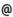## Babes-Bolyai University of Cluj-Napoca Faculty of Mathematics and Computer Science Study Cycle: Graduate SUBJECT

 Code Subject MMA0004 Mathematical Analysis 3
 Section Semester Hours: C+S+L Category Type Mathematics 3 2+2+0 speciality compulsory Mathematics and Computer Science 3 2+2+0 speciality compulsory Applied Mathematics 3 2+2+0 speciality compulsory
 Teaching Staff in Charge
 Assoc.Prof. DIACONU Adrian, Ph.D.,  adiaconumath.ubbcluj.roLect. FINTA Zoltan, Ph.D.,  fzoltanmath.ubbcluj.ro
 Aims Getting to know the classical knowledges of integral calculus of functions of one and several real variables.
 Content I) Line integrals. 1) Vectorfuntions with values in Rn and with bundet variation. Vectorfuntions with the C1 class. The calculation of the total variation for a vectorfuntion with values in Rn and the C1 class. 2) Lines. The length and the lengtfuntion for a lines and theirs expression in the case of the lines with the C1 class. 3) Integration on a line of a real funtion with n real variables. Calculation formulas in special situations. 4) Liniar differential forms and theirs integration. Calculation formulas in special situations. 5) The primitive ao a vectorfuntion and the calculation of the integral of a liniar differential form on a line with the help of the primitive of the generating vectorfuntion. 6) Operations with lines. The conduct of the integral with regard these operations. 7) Theorems regarding the independece of line of the line integrals from a liniar differentil form. Necesary and sufficient conditions for the existence of the primitive of a vectorfunction. 8) Oriented arcs. The independece of the integral of the representative line of the arc. The integral on a oriented arc. II) The Riemann integral of a real function with furthemore variables. 1) The Jordan measure of a set from Rn. Simple sets from R2 and R3 limited by the graphics of continuous functions. 2) The definition of the integrability and the integral of areal function with n real variables. The bounding of the integrabes functions. 3) The Darboux sums in the case of functions with furthemore variables. The theorem of Darboux in this case. 4) The Lebesgue criterion for the Riemann integrability. 5) The additivity with regard to the integration set of the integrability and of the integral. 6) The reduction of the integral for a function with mn variables to the iterate integrals. The Fubinii's theorem. The calculation of the double and the triple integrals through the reduction of iterate integrals. 7) The connection between the integral of a set from R2 and the integral of his orieted border. The Green's formula. 8) The formula of the changing of the variabels for a function with n variabels. The proof in the case of the double integral. Remarcabel cases for the double and the triple integral. III) Surface integrals. 1) Surface parametrisations in R3. The measure of the surface determined through a parametrisation and the integral of a function defined on this surface with regard to the determined measure. 2) Differential forms with the second order and theirs integration on a surface parametrisation. 3) Oriented surface parts in R3. The integral of a differential form with the second order on an oriented surface part. 4) The theorems of Stokes and Gauss- Ostrogradski for the connection between integraltypes. IV) Functions defined through integrals ( integrals with parameter ). 1) Functions types defined through integrals. The uniform convergence of the improper integrals with parameter.
 References 1. BALAZS M.: Analiza matematica, III si IV, Universitate, Cluj-Napoca, 1983,1984 2. BALAZS M., KOLUMBAN I.: Matematikai analizis, Dacia Konivkyado, Kolozsvar-Napoca, 1978 3. BOBOC N.: Analiza matematica, II, Universitate, Bucuresti, 1993 4. BUCUR G., CAMPU E., GAINA S.: Culegere de probleme de calcul diferential si integral, III, Editura tehnica, Bucuresti, 1967 5. COBZAS ST.: Analiza matematica (Calcul diferential), Presa universitara clujeana, Cluj-Napoca, 1997 6. COLOJOARA I.: Analiza matematica, Ed. did. si ped., Bucuresti, 1983 7. DEMIDOVICI B.P.: Culegere de probleme si exercitii de analiza matematica, Ed. tehnica, Bucuresti, 1956 8. SIKORSKI R.: Advanced Calculus, PWN-Polish Scientific Publishiers, Warsawa, 1969 9. WALTER W.: ANALYSIS I, Zweite Aufl. Berlin, Springer-Verlag,1990 10. ***: Analiza matematica, II, Ed. did. si pedag., Bucuresti, 1980 11. HEUSER H.: Lehrbuch der Analysis.Teil 2. 9. Auflage. Stuttgart: B. G. Teubner, 1995.
 Assessment Exam.
 Links: Syllabus for all subjects Romanian version for this subject Rtf format for this subject